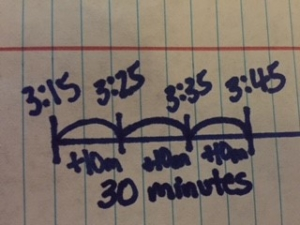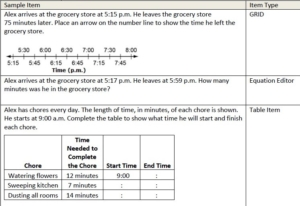To solve story problems involving elapsed time, encourage students to draw open number lines to help students conceptually understand the concept. This could prevent common misconceptions students may have when determining elapsed time.

Make sure to include different types of problems that require students to determine the start time, end time or elapsed time. See the examples of the different types of questions below:

Determine the Elapsed Time:

Riley went to the playground at 3:15. He left the playground at 3:45. How long did Riley spend at the playground?Determine the End Time:

Riley went to the playground at 3:15. He spent 30 minutes at the playground. What time did Riley leave the playground?Determine the Start Time:

Riley spent 30 minutes at the playground. He left the playground at 3:45. What time did Riley get to the playground?It’s helpful to give students similar problems with the same topic, like the ones shown above, to have students sort and determine if they are finding the elapsed time, start time or end time. Encourage students to prove their answer with a number line.

It’s also important to share and discuss different strategies for solving for the elapsed, start or end times. For example, in the problem “Riley went to the playground at 3:15. He left the playground at 3:45. How long did Riley spend at the playground?”

One student below solved it with increments of 10, where another student solved it with increments of 5. Discuss which is most efficient and by showing more than one way, it could help other students have access to the more efficient strategy.Here are some example elapsed time problems from item specifications where students are asked to: determine the end time, determine elapsed time, use a number line, and use a table:For more information on using a number line to determine elapsed time, visit https://learnzillion.com/lesson_plans/9023-solving-elapsed-time-word-problems-to-the-nearest-minute.

For more lesson ideas on using a number line to determine elapsed time, visit NCTM Illuminations at http://illuminations.nctm.org/Lesson.aspx?id=3966.